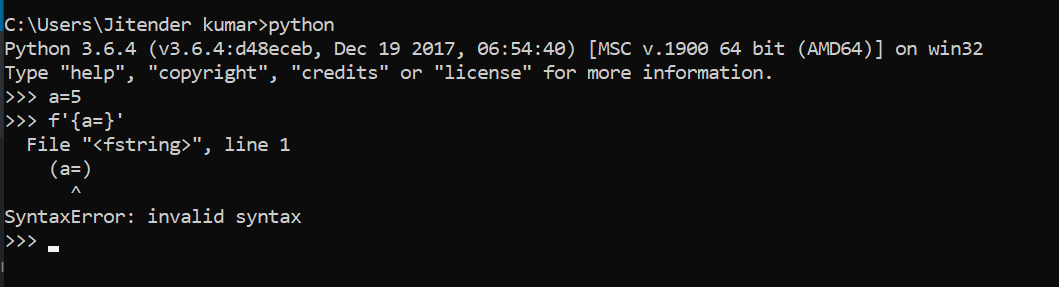# New ‘=’ Operator in Python3.8 f-string

• Last Updated : 03 Jun, 2020

Python have introduced the new = operator in f-string for self documenting the strings in Python 3.8.2 version. Now with the help of this expression we can specify names in the string to get the exact value in the strings despite the position of the variable. Now f-string can be defined as `f'{expr=}'` expression. We can specify any required name in place of expr.

Syntax : `f'{expr=}'`
Return : Return the formatted string.

Attention geek! Strengthen your foundations with the Python Programming Foundation Course and learn the basics.

To begin with, your interview preparations Enhance your Data Structures concepts with the Python DS Course. And to begin with your Machine Learning Journey, join the Machine Learning - Basic Level Course

Note: For older versions of Python this operator will raise the syntax error. See the below image.Example #1 :
In this example we can see that with the help of `f'{expr=}'` expression, we are able to format strings in python by self documenting expressions by using `=` operator.

 `length ``=` `len``(``'GeeksForGeeks'``)`` ` `# Using f'{expr =}' expression``gfg ``=` `f``'The length of GeeksForGeeks is {length =}.'`` ` `print``(gfg)`

Output :

The length of GeeksForGeeks is length=13.

Example #2 :

 `a, b ``=` `5``, ``10`` ` `# Using f'{expr =}' expression``gfg ``=` `f``'Value of {b = } and {a = }.'`` ` `print``(gfg)`

Output :

Value of b = 10 and a = 5.

My Personal Notes arrow_drop_up